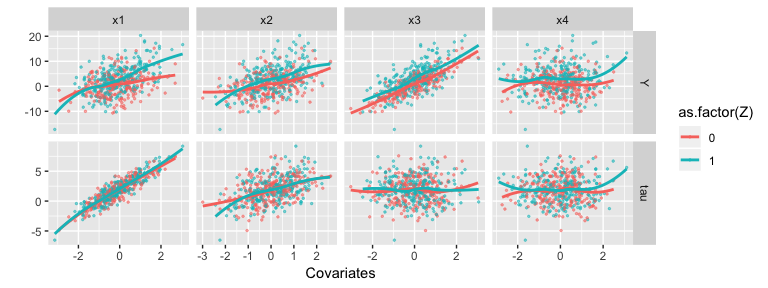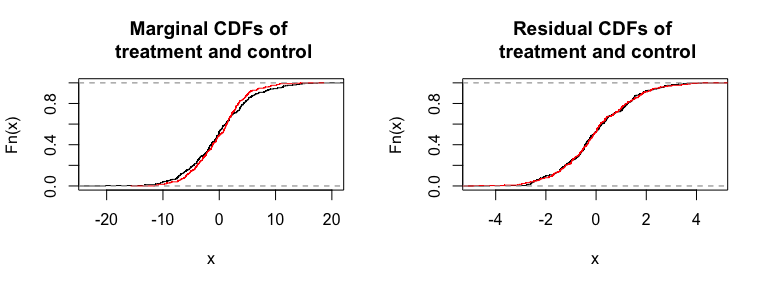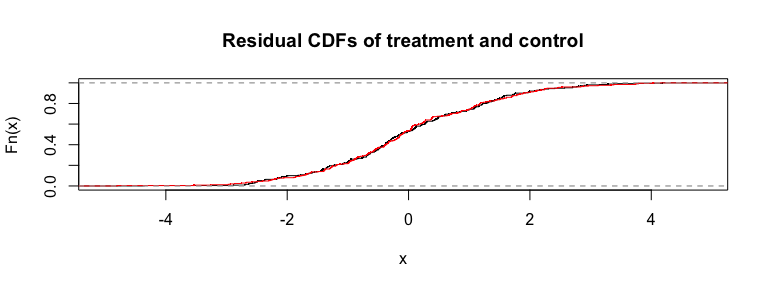# Introduction

This document demonstrates how to perform permutation test inference for heterogeneous treatment effects. We use a simulated dataset to illustrate. This data is provided with the package as . Overall, this vignette illustrates the different tests for ideosyncratic variation one might make using our package, and shows how different forms of covariate adjustment can increase power or target inferential questions differently.

We first load our package along with some other useful packages.

library( mvtnorm )
library( tidyverse )
library( hettx )
data( ToyData )

# The illustrative Dataset

We begin with exploring a toy dataset with 500 observations that we included with the package for illustration. ToyData has an outcome, four covariates, and a treatment indicator. They were generated with the following model: $Y_i(0) = 1 + x_{1i} + 2 x_{2i} + 4x_{3i} + \epsilon_i$ with $$\epsilon_i \sim N( 0, 1^2 )$$. The treatment model is all systematic: $\tau_i = 2 + 2x_{1i} + x_{2i}$ So $$x_1$$ and $$x_2$$ are both predictive of treatment impact. $$x_3$$ also predicts control side variation, and should therefore be useful for increasing precision. $$x_4$$ is useless. $$Y_i(1) = Y_i(0) + \tau_i$$, so there is no ideosyncratic variation if we control for both $$x_1$$ and $$x_2$$.

As we generated these data, we know the true individual treatment effects.

data( ToyData )
head( ToyData )
##           Y Z         x1        x2          x3         x4      tau
## 1 2.2430188 0  0.7635779 0.9415463 -0.50291814 -1.4374786 4.468702
## 2 0.3997241 0 -0.5884278 0.7976774 -0.32835669 -0.7140096 1.620822
## 3 3.5865779 0  0.4881701 0.1335321  0.54933171 -0.7098844 3.109872
## 4 0.9929092 0  1.3796253 0.1786193 -0.23264879 -0.3467998 4.937870
## 5 3.0077115 1  0.1722719 0.2836023 -0.03172434 -2.2100810 2.628146
## 6 2.5547212 0 -0.2978002 0.2678954  0.46776121 -1.6495943 1.672295
td = gather( ToyData, x1, x2, x3, x4, key="X", value="value" )
td = gather( td, Y, tau, key="outcome", value="value2" )
ggplot( td, aes( x=value, y=value2, col=as.factor(Z) ) ) +
facet_grid( outcome ~ X, scales="free" ) +
geom_point( alpha=0.5, size=0.5) +
geom_smooth( method="loess", se=FALSE ) +
labs( x="Covariates", y="" )As the data is simulated, we have the true finite-sample ATE:

mean( ToyData$tau ) ##  1.858165 Before testing, we quickly look at the marginal and residual CDFs of treatment and control. We see heterogeniety at left, but after controlling for observed covariates we have no visibile ideosyncratic heterogeniety left over. par( mfrow=c(1,2) ) ll0 = lm( Y ~ Z, data=ToyData ) plot( ecdf( resid(ll0)[ToyData$Z==1] ), pch=".", main="Marginal CDFs of \n treatment and control")
plot( ecdf( resid(ll0)[ToyData$Z==0] ), pch=".", col="red", add=TRUE ) ll1 = lm( Y ~ Z + x1 + x2 + x3 + x4, data=ToyData ) plot( ecdf( resid(ll1)[ToyData$Z==1] ), pch=".", main="Residual CDFs of \n treatment and control" )
plot( ecdf( resid(ll1)[ToyData$Z==0] ), pch=".", col="red", add=TRUE )A simple linear model should give us our parameters from above, since it is a correct specification in this case. M0 <- lm( Y ~ Z * (x1+x2+x3), data=ToyData ) round( coef( M0 ), digits=1 ) ## (Intercept) Z x1 x2 x3 Z:x1 ## 1.0 2.1 1.1 2.0 4.0 2.0 ## Z:x2 Z:x3 ## 0.9 0.1 # Testing for ideosyncratic treatment effect variation ## Basic case: no covariate adjustment To do our tests, we first must specify some parameters determining the resolution of the grid search and number of permutations at each grid point. Note that these values should be increased for a real analysis. We chose these particular values to reduce computation time for illustration. B <- 20 grid.size = 11 The basic test for ideosyncratic treatment effect variation is as follows (with no adjustment for covariates): tst1 = detect_idiosyncratic( Y ~ Z, data=ToyData, B=B, grid.size = grid.size, verbose=FALSE ) summary( tst1 ) ## ## FRT CI Test for Treatment Effect Heterogeneity ## Call: ## detect_idiosyncratic(formula = Y ~ Z, data = ToyData, B = B, ## grid.size = grid.size, verbose = FALSE) ## ## # observations: 500 ## Test Statistic: SKS.stat ## Grid: 11 points tested over range of 0.347343 to 3.936448 with 20 repititions/point ## Estimated ATE (grid center): 2.142 ## gamma (for CI for grid): 0.000100 ## ## Statistic P-Value (Sweep) P-Value (Plug-In) ## 0.08 0.1001 0.1001 ## CI for p-value (monte carlo error) = 0.000100 - 0.317083 # Adjusting for covariates We can increase the power by adjusting for covariates. Please note that we do not include any interaction terms at this point. We specify a control.formula which will be used to generate a matrix to hand to the linear regression function. This will convert factors to dummy variables as needed. tst2 = detect_idiosyncratic( Y ~ Z, data=ToyData, control.formula = ~ x1 + x2 + x3 + x4, B=B, test.stat="SKS.stat.cov", verbose=FALSE ) summary( tst2 ) ## ## FRT CI Test for Treatment Effect Heterogeneity ## Call: ## detect_idiosyncratic(formula = Y ~ Z, data = ToyData, control.formula = ~x1 + ## x2 + x3 + x4, test.stat = "SKS.stat.cov", B = B, verbose = FALSE) ## ## # observations: 500 ## Test Statistic: SKS.stat.cov ## Grid: 151 points tested over range of 1.441206 to 2.454906 with 20 repititions/point ## Estimated ATE (grid center): 1.948 ## gamma (for CI for grid): 0.000100 ## ## Statistic P-Value (Sweep) P-Value (Plug-In) ## 0.232 1e-04 1e-04 ## CI for p-value (monte carlo error) = 0.000100 - 0.168533 Let’s explore how the results might differ when non-treatment covariates are used. tst2b = detect_idiosyncratic( Y ~ Z, data=ToyData, control.formula = ~ x3 + x4, B=B, test.stat="SKS.stat.cov", verbose=FALSE ) summary( tst2b ) ## ## FRT CI Test for Treatment Effect Heterogeneity ## Call: ## detect_idiosyncratic(formula = Y ~ Z, data = ToyData, control.formula = ~x3 + ## x4, test.stat = "SKS.stat.cov", B = B, verbose = FALSE) ## ## # observations: 500 ## Test Statistic: SKS.stat.cov ## Grid: 151 points tested over range of 1.047639 to 3.444855 with 20 repititions/point ## Estimated ATE (grid center): 2.246 ## gamma (for CI for grid): 0.000100 ## ## Statistic P-Value (Sweep) P-Value (Plug-In) ## 0.148 1e-04 1e-04 ## CI for p-value (monte carlo error) = 0.000100 - 0.168533 We can compare to when we correct for some useless covariates not related to outcome. N = nrow(ToyData) ToyData$x4 = rnorm( N )
tst1b = detect_idiosyncratic( Y ~ Z, data=ToyData, control.formula = ~ x4, B=B,
test.stat="SKS.stat.cov", verbose=FALSE )
summary( tst1b )
##
## FRT CI Test for Treatment Effect Heterogeneity
## Call:
## detect_idiosyncratic(formula = Y ~ Z, data = ToyData, control.formula = ~x4,
##     test.stat = "SKS.stat.cov", B = B, verbose = FALSE)
##
## # observations: 500
## Test Statistic: SKS.stat.cov
## Grid: 151 points tested over range of 0.349867 to 3.940811 with 20 repititions/point
## Estimated ATE (grid center): 2.145
## gamma (for CI for grid): 0.000100
##
##  Statistic P-Value (Sweep) P-Value (Plug-In)
##      0.084          0.0501            0.0501
##  CI for p-value (monte carlo error) = 0.000100 - 0.248833

## Ideosyncratic variation beyond systematic variation

To test for ideosyncratic variation beyond systematic, we pass an interaction.formula to the method.

We first test for ideosyncratic variation beyond x1 (and we should get high $$p$$-value).

B = 20

tst3a1 = detect_idiosyncratic( Y ~ Z, data=ToyData, interaction.formula = ~ x1, B=B,
test.stat="SKS.stat.int.cov", verbose=FALSE )
summary( tst3a1 )
##
## FRT CI Test for Tx Effect Heterogeneity Beyond a Systematic Model
## Call:
## detect_idiosyncratic(formula = Y ~ Z, data = ToyData, interaction.formula = ~x1,
##     test.stat = "SKS.stat.int.cov", B = B, verbose = FALSE)
##
## # observations: 500
## Test Statistic: SKS.stat.int.cov
## Grid: 151 points tested with 20 repititions/point
## Grid range:
##             Z       Z:W
##      1.126009 0.9634314
##      3.172938 3.3136498
## gamma (for CI for grid): 0.000100
##
##  Statistic P-Value (Sweep) P-Value (Plug-In)
##      0.072          0.3501            0.1501

Include additional terms to increase power. We are correcting for x3 and x4.

tst3a2 <- detect_idiosyncratic( Y ~ Z, data=ToyData,
interaction.formula = ~ x1,
control.formula = ~ x3 + x4,
B=B, test.stat="SKS.stat.int.cov",
verbose=FALSE )
summary( tst3a2 )
##
## FRT CI Test for Tx Effect Heterogeneity Beyond a Systematic Model
## Call:
## detect_idiosyncratic(formula = Y ~ Z, data = ToyData, interaction.formula = ~x1,
##     control.formula = ~x3 + x4, test.stat = "SKS.stat.int.cov",
##     B = B, verbose = FALSE)
##
## # observations: 500
## Test Statistic: SKS.stat.int.cov
## Grid: 151 points tested with 20 repititions/point
## Grid range:
##             Z      Z:W
##      1.718816 1.564162
##      2.841292 2.832136
## gamma (for CI for grid): 0.000100
##
##  Statistic P-Value (Sweep) P-Value (Plug-In)
##      0.128           1e-04             1e-04

For comparison, we next include all terms.

tst3a2b <- detect_idiosyncratic( Y ~ Z, data=ToyData, control.formula = ~ x2 + x3 + x4,
interaction.formula = ~ x1, B=B, test.stat="SKS.stat.int.cov",
verbose=FALSE )
summary( tst3a2b )
##
## FRT CI Test for Tx Effect Heterogeneity Beyond a Systematic Model
## Call:
## detect_idiosyncratic(formula = Y ~ Z, data = ToyData, interaction.formula = ~x1,
##     control.formula = ~x2 + x3 + x4, test.stat = "SKS.stat.int.cov",
##     B = B, verbose = FALSE)
##
## # observations: 500
## Test Statistic: SKS.stat.int.cov
## Grid: 151 points tested with 20 repititions/point
## Grid range:
##             Z      Z:W
##      1.902294 1.810105
##      2.400747 2.276669
## gamma (for CI for grid): 0.000100
##
##  Statistic P-Value (Sweep) P-Value (Plug-In)
##      0.116           1e-04             1e-04

### Testing for ideosyncratic variation.

Now correct for the other covariates as well, but still have correct heterogeneous treatment model. Note that you should still get high $$p$$-value.

Start testing for variation beyond x1 and x2.

tst3b <- detect_idiosyncratic( Y ~ Z, data=ToyData,
interaction.formula = ~ x1 + x2, B=B, test.stat="SKS.stat.int.cov",
verbose=FALSE )
summary( tst3b )
##
## FRT CI Test for Tx Effect Heterogeneity Beyond a Systematic Model
## Call:
## detect_idiosyncratic(formula = Y ~ Z, data = ToyData, interaction.formula = ~x1 +
##     x2, test.stat = "SKS.stat.int.cov", B = B, verbose = FALSE)
##
## # observations: 500
## Test Statistic: SKS.stat.int.cov
## Grid: 151 points tested with 20 repititions/point
## Grid range:
##             Z     Z:Wx1      Z:Wx2
##      1.203125 0.9549551 -0.2207353
##      2.702591 2.8468977  1.9497749
## gamma (for CI for grid): 0.000100
##
##  Statistic P-Value (Sweep) P-Value (Plug-In)
##      0.044          1.0001            1.0001

Continue to test for ideosyncratic variation beyond x1 and x2, adjusting for x3 and x4. Note that you should still get high $$p$$-value.

tst3c <- detect_idiosyncratic( Y ~ Z, data=ToyData,
interaction.formula = ~ x1 + x2,
control.formula = ~ x3 + x4,
B=B, test.stat="SKS.stat.int.cov",
verbose=FALSE )
summary( tst3c )
##
## FRT CI Test for Tx Effect Heterogeneity Beyond a Systematic Model
## Call:
## detect_idiosyncratic(formula = Y ~ Z, data = ToyData, interaction.formula = ~x1 +
##     x2, control.formula = ~x3 + x4, test.stat = "SKS.stat.int.cov",
##     B = B, verbose = FALSE)
##
## # observations: 500
## Test Statistic: SKS.stat.int.cov
## Grid: 151 points tested with 20 repititions/point
## Grid range:
##             Z    Z:Wx1     Z:Wx2
##      1.829418 1.792071 0.7191368
##      2.323732 2.256887 1.1672708
## gamma (for CI for grid): 0.000100
##
##  Statistic P-Value (Sweep) P-Value (Plug-In)
##      0.072          0.4001            0.2501

Finally, test for ideosyncratic variation beyond all covariates, even irrelevant ones. Again, you should expect to get high $$p$$-value.

tst3d <- detect_idiosyncratic( Y ~ Z, data=ToyData,
interaction.formula = ~ x1 + x2 + x3 + x4,
B=B, test.stat="SKS.stat.int.cov",
verbose=FALSE )
summary( tst3d )
##
## FRT CI Test for Tx Effect Heterogeneity Beyond a Systematic Model
## Call:
## detect_idiosyncratic(formula = Y ~ Z, data = ToyData, interaction.formula = ~x1 +
##     x2 + x3 + x4, test.stat = "SKS.stat.int.cov", B = B, verbose = FALSE)
##
## # observations: 500
## Test Statistic: SKS.stat.int.cov
## Grid: 151 points tested with 20 repititions/point
## Grid range:
##             Z    Z:Wx1     Z:Wx2       Z:Wx3      Z:Wx4
##      1.815264 1.738528 0.6923846 -0.08096648 -0.1257144
##      2.293039 2.217095 1.1560266  0.35426596  0.3737942
## gamma (for CI for grid): 0.000100
##
##  Statistic P-Value (Sweep) P-Value (Plug-In)
##      0.064          0.6001            0.4001

# Comparing the tests

We can easily compare the results by simultaneously displaying the outputs from all tested models by using the get.p.value() method. This method extracts some core summary statistics:

get.p.value( tst1b )
##   p.value     min.p     max.p      plug
## 0.0501000 0.0000000 0.2487328 0.0501000

We can thus collect all our models from above, and make a dataframe of the overall results:

tests = list( no_cov=tst1, useless_cov=tst1b, all_covariates=tst2,
non_tx_covariates_only=tst2b, het_beyond_x1 = tst3a1,
het_beyond_x1_with_x3_x4_cov=tst3a2, het_beyond_x1_with_all_cov=tst3a2,
het_beyond_x1_x2=tst3b,
het_beyond_x1_x2_with_cov=tst3c, het_beyond_all=tst3d )

agg.res = purrr::map( tests, get.p.value  ) %>%
purrr::map( as.list )
agg.res = bind_rows( agg.res, .id = "test" )
agg.res
## # A tibble: 10 x 5
##    test                         p.value  min.p max.p   plug
##    <chr>                          <dbl>  <dbl> <dbl>  <dbl>
##  1 no_cov                        0.100  0      0.317 0.100
##  2 useless_cov                   0.0501 0      0.249 0.0501
##  3 all_covariates                0.0001 0      0.168 0.0001
##  4 non_tx_covariates_only        0.0001 0      0.168 0.0001
##  5 het_beyond_x1                 0.350  0.0123 0.592 0.150
##  6 het_beyond_x1_with_x3_x4_cov  0.0001 0      0.168 0.0001
##  7 het_beyond_x1_with_all_cov    0.0001 0      0.168 0.0001
##  8 het_beyond_x1_x2              1.00   0.509  1     1.00
##  9 het_beyond_x1_x2_with_cov     0.400  0.0123 0.639 0.250
## 10 het_beyond_all                0.600  0.0123 0.809 0.400

# Cautionary Tale: A linear model with no treatment interaction.

Let’s fit a model that allows for no systematic treatment impact heterogeniety. This means that all variation would have to be considered ideosyncratic. The key, however, is we control for covariates to increase precision.

ll1 = lm( Y ~ Z + x1 + x2 + x3 + x4, data=ToyData )
print( summary( ll1 ) )
##
## Call:
## lm(formula = Y ~ Z + x1 + x2 + x3 + x4, data = ToyData)
##
## Residuals:
##     Min      1Q  Median      3Q     Max
## -4.8012 -0.9466 -0.1229  1.0427  4.1802
##
## Coefficients:
##             Estimate Std. Error t value Pr(>|t|)
## (Intercept)  1.06724    0.09224  11.570   <2e-16 ***
## Z            1.94719    0.13033  14.940   <2e-16 ***
## x1           2.11511    0.06758  31.296   <2e-16 ***
## x2           2.43665    0.06915  35.236   <2e-16 ***
## x3           3.97763    0.06681  59.541   <2e-16 ***
## x4          -0.04612    0.06639  -0.695    0.488
## ---
## Signif. codes:  0 '***' 0.001 '**' 0.01 '*' 0.05 '.' 0.1 ' ' 1
##
## Residual standard error: 1.455 on 494 degrees of freedom
## Multiple R-squared:  0.9243, Adjusted R-squared:  0.9236
## F-statistic:  1207 on 5 and 494 DF,  p-value: < 2.2e-16

The estimated ATE is close to the truth, as expected considering the random assignment.

Next plot residual CDFS of treatment and control groups.

plot( ecdf( resid(ll1)[ToyData$Z==1] ), pch=".", main="Residual CDFs of treatment and control" ) plot( ecdf( resid(ll1)[ToyData$Z==0] ), pch=".", col="red", add=TRUE )Note the residual ECDFs from above are quite aligned. The Tx effect variation has been picked up by main effects which means we would not detect ideosyncratic variation even though there is such variation. In other words, the treatment variation has been distributed across the residuals of the control group as well as treatment, and thus when we compare the distributions they are the same. This is why the choice of test statistic is delicate, and why for the covariate adjusted SKS statistic, we need to fit the model on the control units only, and then extract the residuals.

# A simple variance ratio test

We also offer an adjusted variance ratio test:

variance.ratio.test( ToyData$Y, ToyData$Z )
##         pvalue     var1    var0    ratio log.ratio    asy.se        z
## 1 0.0004993163 32.53071 20.6582 1.574711  0.454072 0.1379776 3.290912

This does not use permutation inference.

# The variety of test statistics

We offer several test statistics one might use. We also have a method to print out some info on what is available:

test.stat.info()
##
## ## -----------------------------------------------------
## ## Available test statistics for detect.idiosyncratic():
## ## -----------------------------------------------------
## Test statistics when not adjusting for covariates or specifying interactions:
##  1) KS.stat - Calculate classic (not shifted) KS statistic. If tau passed, Y1 will be shifted by tau.
##  2) SKS.stat - Shifted KS statistic. Calculate KS distance between Y0 and Y1 shfited by tau.
##  3) rq.stat - Classic KS statistic via quantile regression with covariates
##
## Test statistics when adjusting for covariates but not specifying interactions:
##  1) SKS.stat.cov.pool - Shifted KS statistic with covariates to increase precision.
##  2) SKS.stat.cov - Shifted KS statistic with covariates with model for outcomes calculated on control group only.
##     This avoids 'splitting' the treatment variation between treatment and control groups. We recommend this method
##     over the 'pool' method in SKS.stat.cov.pool.
##  3) SKS.stat.cov.rq - Shifted KS statistic via quantile regression with covariates.
##  4) rq.stat.cond.cov - KS statistic via quantile regression with covariates. Conditional approach; see Koenker
##      and Xiao (2002) for more information.
##  5) rq.stat.uncond.cov - KS statistic via quantile regression with covariates. Unconditional approach; see
##      Firpo (2007) for more information.
##
## Test statistics when specifying interactions, with or without covariate adjustment:
##  1) SKS.stat.int.cov.pool - Shifted KS statistic with a linear treatment effect model and optional covariate
##     adjustment. This will attempt to remove any systematic variation corresponding to the specified interaction
##     model and then return an SKS statistic on the residuals to measure any variation 'left over'. This is the
##     test statistic used in Ding, Feller, and Miratrix (2016), JRSS-B.
##  2) SKS.stat.int.cov - Similar to SKS.stat.int.cov.pool, but this method first adjusts for baseline and then
##     models treatment effects on the residuals to not split treatment effects. We recommend this method over
##     the 'pool' method in SKS.stat.int.cov.pool.
##
## Test statistics when specifying interactions, without covariate adjustment:
##  1) WSKS.t - Calculates the shifted KS statistic within each group specified in the interaction model, and
##     then aggregates together as a weighted average. Should be used when the interaction model is a single
##     categorical or factor covariate.
##  2) SKS.pool.t - Subtract off group level treatment effect estimates and then look at KS statistic on residuals.
##     Should be used when the interaction model is a single categorical or factor covariate.

`